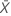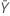# Methods and formulas for Test mean - reference mean for 2-Sample Equivalence Test

The following methods and formulas are used for testing the difference between the test mean and the reference mean.

## Difference (D)

### Notation

TermDescription
DDifferenceTest meanReference mean

## Means and standard deviations

The mean of the test sample,, is given by:

The mean of the reference sample,, is given by:The standard deviation of the test sample, S1, is given by:The standard deviation of the reference sample, S2, is given by:### Notation

TermDescription
X iObservations from the test sample, with i = 1, ..., n1
Y iObservations from the reference sample, with i = 1, ..., n2
n1Number of observations in the test sample
n2Number of observations in the reference sample

## Standard error of the difference (SE)

### Do not assume equal variances (default)

By default, Minitab uses the following formula to calculate the standard error of the difference, SE:

### Assume equal variances

If you select the option to assume equal variances, then Minitab calculates the pooled standard deviation, Sp, and the standard error of the difference, SE, using the following formulas:### Notation

TermDescription
S1Standard deviation of the test sample
n1Number of observations in the test sample
S2Standard deviation of the reference sample
n2Number of observations in the reference sample
SpPooled standard deviation

## Equivalence limits

Let k1 be the value that you specify for the lower limit and k2 be the value that you specify for the upper limit. By default, the lower equivalence limit, δ1, is given by:and the upper equivalence limit, δ2, is given by:## Degrees of freedom (DF)

### Do not assume equal variances (default)

By default, the degrees of freedom for the test, v, are given by the following formula:

Minitab displays v rounded down to the nearest integer.

### Assume equal variances

If you select the option to assume equal variances, then Minitab calculates the degrees of freedom as follows:### Notation

TermDescription
S1Standard deviation of the test sample
n1Number of observations in the test sample
S2Standard deviation of the reference sample
n2Number of observations in the reference sample

## Confidence interval

### 100(1-α)% CI

By default, Minitab uses the following formula to calculate the 100(1 – α)% confidence interval (CI) for equivalence:

CI = [min(C, Dl), max(C, Du)]

where:

### 100(1-2α)% CI

If you select the option to use the 100(1 – 2α)% CI, then the CI is given by the following formula:

CI = [Dl, Du]

### One-sided intervals

For a hypotheses of Test mean > reference mean or Test mean - reference mean > lower limit, the 100(1 – α)% lower bound is equal to DL.

For a hypothesis of Test mean < reference mean or Test mean - reference mean < upper limit, the 100(1 – α)% upper bound is equal to DU.

### Notation

TermDescription
DDifference between the test mean and the reference mean
SEStandard error
δ1Lower equivalence limit
δ2Upper equivalence limit
vDegrees of freedom
αThe significance level for the test (alpha)
t1-α, vUpper 1 – α critical value for a t-distribution with v degrees of freedom

## T-values

Let t1 be the t-value for the hypothesis,, and let t2 be the t-value for the hypothesis,, whereis the difference between the mean of the test population and the mean of the reference population. By default, the t-values are calculated as follows:

For a hypothesis of Test mean > reference mean, δ1 = 0.

For a hypothesis of Test mean < reference mean, δ 2 = 0.

### Notation

TermDescription
DDifference between the sample test mean and the sample reference mean
SEStandard error of the difference
δ1Lower equivalence limit
δ2Upper equivalence limit

## P-values

The probability, PH0, for each null hypothesis (H0) is given by the following:
H0 P-Value### Notation

TermDescriptionUnknown difference between the mean of the test population and the mean of the reference population
δ1Lower equivalence limit
δ2Upper equivalence limit
vDegrees of freedom
Tt-distribution with v degrees of freedom
t1 t-value for the hypothesist2 t-value for the hypothesis###### Note

For information on how the t-values are calculated, see the section on t-values.

By using this site you agree to the use of cookies for analytics and personalized content.  Read our policy